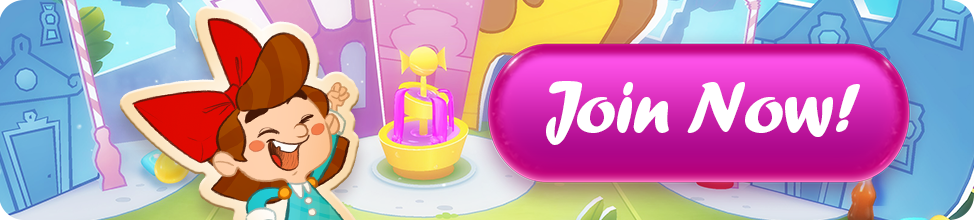# (FINISHED) Win GOLD BARS - Balance the scale!

• @plushytoysfan - that is a contest for a different game and no one has to use the spoiler.

·
• 8 + 1 = 3 + 6

5 + 5 = 4 + 6

3 + 7 = 1 + 9

2 + 6 = 5 + 3

3 + 9 = 6 + 6

·
• This is a spoiler message. My answers are

8+3 1+6

5+5 4+6

7+3 1+9

5+2 3+6

6+3 6+9

·
• Hi @Elsa

1. 8 + 1, 3 + 6

2. 5 + 5, 4 + 6

3. 3 + 7, 1 + 9

4. 2 + 6, 5 + 3

5. 3 + 9, 6 + 6

·
• More answers still rolling in

·
• I absolutely love maths or any puzzle to get my brain working, thank you for the challenge 😀 my answer is...

1) 8+1=9

6+3=9

2) 5+5=10

4+6=10

3) 7+3=10

1+9=10

4) 6+2=8

5+3=8

5) 6+6=12

9+3=12

·
• 8+1 & 3+6

5+5 & 4+6

3+7 & 1+9

2+6 & 3+5

3+9 & 6+6

·
• edited January 12

How do you get the spoiler box?

·

• 3+6 – 8+1

5+5 – 4+6

3+7 – 1+9

2+6 – 5+3

3+9 – 6+6

·
• Posts: 34,525 Community Hub Moderator

Hi @PattyRae welcome to our community. This is how to find the spoiler box.

·
• @Elsa thanks for this I equally didn't know myself

·
• 3+6 - 8+1

5+5 - 4+6

3+7 - 1+9

6+2 - 3+5

3+9 - 6+6

·
• I will try to balance this scale. My responses on SPOILER. (if I can post)

1 - 8+1 6+3

2 - 5+5 4+6

3 - 3+7 1+9

4 - 5+3 6+2

5 - 6+6 9+3

·
• 8,1 + 6,3

5,5 + 4,6

1,9 + 7,3

6,2 + 5,3

6,6 + 3,9

Thanks for the opportunity. Do you let us know if we are wrong?

·
• edited January 14

6+3. 8+1; 5+5. 6+4; 7+3. 9+1; 6+2. 5+3 Keep on popping soda!

Pop pop pop onto the next level

·